# Segment tree

The segment tree is a highly versatile data structure, based upon the divide-and-conquer paradigm, which can be thought of as a tree of intervals of an underlying array, constructed so that queries on ranges of the array as well as modifications to the array's elements may be efficiently performed.

## Motivation

One of the most common applications of the segment tree is the solution to the range minimum query problem. In this problem, we are given some array and repeatedly asked to find the minimum value within some specified range of indices. For example, if we are given the array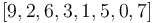$[9,2,6,3,1,5,0,7]$, we might be asked for the minimum element between the third and the sixth, inclusive, which would be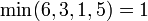$\min(6,3,1,5) = 1$. Then, another query might ask for the minimum element between the first and third, inclusive, and we would answer 2, and so on. Various solutions to this problem are discussed in the range minimum query article, but the segment tree is often the most appropriate choice, especially when modification instructions are interspersed with the queries. For the sake of brevity, we shall focus for several following sections on the type of segment tree designed to answer the range minimum query without explicitly re-stating each time that we are doing so. Bear in mind, however, that other types of segment tree exist, which are discussed later in the article.

### The divide-and-conquer solution

The divide-and-conquer solution would be as follows:

• If the range contains one element, that element itself is trivially the minimum within that range.
• Otherwise, divide the range into two smaller ranges, each approximately half the size of the original, and find their respective minima. The minimum for the original range is then the smaller of the two minima of the sub-ranges.

Hence, if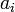$a_i$ denotes the$i$th element in the array, finding the minimum could be encoded as the following recursive function: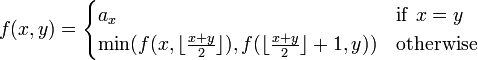$\displaystyle f(x,y) = \begin{cases} a_x & \mathrm{if\ } x = y \\ \min(f(x,\lfloor\frac{x+y}{2}\rfloor),f(\lfloor\frac{x+y}{2}\rfloor+1,y)) & \mathrm{otherwise} \\ \end{cases}$

assuming that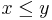$x \le y$.
Hence, for example, the first query from the previous section would be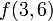$f(3,6)$ and it would be recursively evaluated as$\min(f(3,4),f(5,6))$.

## Structure

Suppose that we use the function defined above to evaluate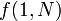$f(1,N)$, where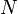$N$ is the number of elements in the array. When$N$ is large, this recursive call has two "children", one of which is the recursive call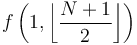$f\left(1,\left\lfloor\frac{N+1}{2}\right\rfloor\right)$, and the other one of which is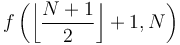$f\left(\left\lfloor\frac{N+1}{2}\right\rfloor+1,N\right)$. Each of these children will then have two children of its own, and so on, down until the base case is reached. If we represent these recursive calls with a tree structure, the call$f(1,N)$ would be the root, it would have two children, each child would have two more children, and so on; the base cases would be the leaves of the tree. We are now ready to specify the structure of the segment tree:

• it is a binary tree which represents some underlying array;
• each node is associated with some interval of the array and contains the value(s) of one or more functions of the elements in that interval;
• the root node is associated with the entire array (i.e. the interval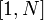$[1,N]$);
• each leaf is associated with an individual element;
• each non-leaf node has two children whose associated intervals are disjoint, and the union of the intervals associated with the two children is the interval associated with the parent;
• each child's interval has approximately half the size of the parent's interval;
• the data stored in each non-leaf node is not only a function of the elements in its associated interval but also a function of the data stored in its children.

That is, the structure of the segment tree is exactly that of the recursive call tree of the function$f(1,N)$ defined above (up to some minor details, such as whether the middle element of an interval of odd size belongs to the left child interval or the right).
Hence, for example, the root node of the array$[9,2,6,3,1,5,0,7]$ would contain the number 0: the minimum of the entire array. Its left child would contain the minimum of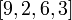$[9,2,6,3]$, that is, 2, and the right would contain the minimum of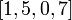$[1,5,0,7]$, that is, 0. Evidently, the datum stored in the root node, 0, is the minimum of the data stored at its immediate children, 0 and 2. Similarly, the datum 2 stored in the left child is the minimum of the data stored at its immediate children, 2 and 3 (which are the minima in$[9,2]$ and$[6,3]$, respectively). Each individual element in the array has a leaf node, which merely contains that element itself. (The minimum of a range containing a single element is, of course, the element itself.)

## Operations

There are three fundamental operations on a segment tree.

### Construction

To construct a segment tree is to initialize it so that it represents some array; we can query and modify this array later but first we need to construct a valid segment tree. We can construct a segment tree either top-down or bottom-up. Top-down construction is recursive; we attempt to fill in the root node, which leads to a recursive call on each of the two children, and so on; the base cases are the leaf nodes, which may be immediately filled in with the corresponding values from the array. After the two recursive calls made on behalf of a non-leaf node return, that node's value is set to the minimum of the values stored at the children. Bottom-up construction is left as an exercise for the reader; the difference in speed is likely to be negligible.

### Update0 is changed to 8 in the segment tree above. Yellow nodes are those on the path from the root to the modified leaf; they are recursively traversed and their data are modified as the update occurs.

To update a segment tree is to modify the value of one element in the underlying array. To do so, we first modify the corresponding leaf node. The other leaf nodes are not affected, since each leaf node is associated only with an individual element. The modified node's parent is affected, since its associated interval contains the modified element, and so is the grandparent, and so on up to the root, but no other nodes are affected. To execute a top-down update, we begin by requesting an update of the root; this leads to a recursive call of one of the two children --- the one whose associated interval contains the modified element. (The other child and its subtree are unaffected.) The same is done recursively for the child; the base case is the leaf node associated with the element to update. After a recursive call has completed, the value in a non-leaf node is re-evaluated as the minimum of the values at its two children. Updating a segment tree bottom-up is possible too and is again left as an exercise for the reader.

### Query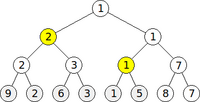Only the two nodes marked in yellow must be accessed to find the minimum of the elements corresponding to the leaves marked in grey.

To query a segment tree is to use it to determine a function of a range in the underlying array (in this case, the minimum element of that range). The execution of a query is more complex than the execution of an update and will be illustrated by example. Suppose we wish to know the minimum element between the first and sixth, inclusive. We shall represent this query as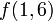$f(1,6)$. Each node in the segment tree contains the minimum in some interval: for example, the root node contains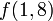$f(1,8)$, its left child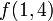$f(1,4)$, its right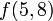$f(5,8)$, and so on, with each leaf containing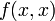$f(x,x)$ for some$x$. There is no node that contains$f(1,6)$, but we notice that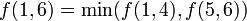$f(1,6) = \min(f(1,4),f(5,6))$, and that there are nodes in the segment tree containing those two values (shown in yellow). (This expression for$f(1,6)$ is not the one given by the definition of$f$, but it is fairly clear that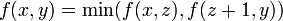$f(x,y) = \min(f(x,z),f(z+1,y))$ where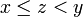$x \le z < y$, regardless of the actual value of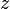$z$.)
When querying a segment tree, therefore, we select a subset of the nodes with the property that the union of their sets of descendants is exactly the interval whose minimum we seek. To do so, we start at the root and recurse over nodes whose corresponding intervals have at least one element in common with the query interval. Hence, in our example of$f(1,6)$, we notice that both the left and right subtrees contain descendants that contain elements in the query interval; hence, we recurse on both. The left child of the root serves as a base case, since its interval is contained entirely within the query interval; hence it is chosen (marked in yellow). At the right child of the root, we notice that its left child has descendants in the query interval, but its right does not; hence we recurse on the former and not the latter. The former is now another base case, and it too is chosen and marked in yellow. The recursion has now terminated, and the desired minimum is the minimum of the nodes that have been chosen.

## Analysis

Some important performance bounds for segment trees are as follows:

### Space

A node whose depth is$d$ is associated with an interval of size no greater than$\left\lceil{\frac{N}{2^d}}\right\rceil$. This is easily proven by induction on$d$. We therefore see that all nodes at depth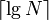$\lceil\lg N \rceil$ are responsible for no more than one element: they are leaves. Hence, a segment tree is a fully balanced binary tree; it has the minimum possible height for the number of nodes it contains. Because of this, we may store the tree as a breadth-first traversal of its nodes, where the left child is (by convention) explored before the right. The segment tree above for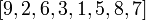$[9,2,6,3,1,5,8,7]$, then, would be stored as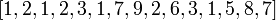$[1,2,1,2,3,1,7,9,2,6,3,1,5,8,7]$. Suppose the root is stored at index 1. Then, if a non-leaf node has index$i$, its left child will be stored at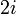$2i$ and its right at$2i+1$. Note that there will be some wasted space on the lowest level of the tree; this however is generally acceptable. A binary tree with a depth of$\lceil\lg N\rceil$ may have up to$2^{\lceil\lg N\rceil + 1}-1$ elements, so some mathematical analysis shows that a segment tree on an array of$N$ elements requires an array of no more than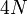$4N$ elements for its own storage in this manner. Asymptotically, a segment tree uses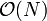$\mathcal{O}(N)$ space.

### Time

#### Construction

Construction requires a constant number of operations on each node of the segment tree; as there are$\mathcal{O}(N)$ nodes, the construction takes linear time. You will notice that this is faster than doing$N$ separate update operations.

#### Update

A constant number of operations is performed for each node on the path from the root to the leaf associated with the modified element. The number of nodes on this path is bounded by the height of the tree; hence, per the conclusions in the Space section above, the time required for an update is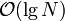$\mathcal{O}(\lg N)$.

#### Query

Consider the set of selected nodes (marked in yellow in the illustration). It is possible, in the case of a query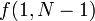$f(1,N-1)$, that there are logarithmically many. Can there be more? The answer is no. The simplest way of proving this, perhaps, is by exhibiting an algorithm for selecting the nodes that terminates within$\mathcal{O}(\lg N)$ steps; this is the non-recursive algorithm alluded to earlier. So a query takes$\mathcal{O}(\lg N)$ time. The proof that the recursive version also takes$\mathcal{O}(\lg N)$ time is left as an exercise for the reader.

## Implementation

object rmq_segtree
private function build_rec(node,begin,end,a[])
if begin = end
A[node] = a[begin];
else
let mid = floor((begin+end)/2)
build_rec(2*node,begin,mid,a[])
build_rec(2*node+1,mid+1,end,a[])
A[node] = min(A[2*node],A[2*node+1])
private function update_rec(node,begin,end,pos,val)
if begin = end
A[node] = val
else
let mid=floor((begin+end)/2)
if pos<=mid
update_rec(2*node,begin,mid,pos,val)
else
update_rec(2*node+1,mid+1,end,pos,val)
A[node] = min(A[2*node],A[2*node+1])
private function query_rec(node,t_begin,t_end,a_begin,a_end)
if t_begin>=a_begin AND t_end<=a_end
return A[node]
else
let mid = floor((t_begin+t_end)/2)
let res = ∞
if mid>=a_begin AND t_begin<=a_end
res = min(res,query_rec(2*node,t_begin,mid,a_begin,a_end))
if t_end>=a_begin AND mid+1<=a_end
res = min(res,query_rec(2*node+1,mid+1,t_end,a_begin,a_end))
return res
function construct(size,a[1..size])
let N = size
let A be an array that can hold at least 4N elements
build_rec(1,1,N,a)
function update(pos,val)
update_rec(1,1,N,pos,val)
function query(begin,end)
return query_rec(1,1,N,begin,end)


## Variations

The segment tree can be adapted to retrieve not only the minimum element in a range but also various other functions. Here are some examples taken from otherwise difficult contest problems:

### Maximum

Analogously to the minimum: all min operations replaced by max.

### Sum or product

Each non-leaf node contains the sum of the values at its children. For example, in the pseudocode above, all min operations would be replaced by addition operations. However, in the case of sums, the segment tree is superseded by the binary indexed tree, which is smaller (in terms of space), faster, and simpler to code. Product can be implemented in the same way, with multiplication in place of the addition.

### Maximum/minimum prefix/suffix sum

A prefix of a range consists of the first$k$ elements in that range ($k$ may be zero); a suffix is analogously defined for the last$k$ elements. The maximal sum prefix of a range is the prefix with maximal sum (where the empty range has sum zero). That maximal sum is known as the maximum prefix sum (minimum prefix sum defined analogously). We would like to be able to query maximum prefix sum in an interval efficiently. For example, the maximum prefix sum in$[1,-2,3,-4]$ is 2, the sum of the prefix$[1,-2,3]$; no other prefix has a higher sum.

To solve this problem with a segment tree, we let each node store two functions of its associated interval: the maximum prefix sum and the total sum (of all elements in the interval). The total sum at a non-leaf node is the sum of the total sums at the two children. To find the max prefix sum at a non-leaf node, we notice that the maximal sum prefix ends either within the left child interval or within the right; in the former case, we simply take the maximal prefix sum from the left child, whereas in the latter we add the total sum from the left child and the maximal prefix sum from the right. The maximum of these two values then gives our answer. The query operation is similar, but with potentially more than two adjacent intervals (in which the optimal location for the end of the maximal sum prefix might lie in any one of them).

### Maximum/minimum subvector sum

This problem asks for the maximal sum of any sub-interval of a given interval. That is, it is similar to the max prefix sum problem explained in the previous section, but the beginning of the sub-interval is not anchored to the beginning of the given interval. (The maximal subvector sum in$[1,-2,3,-4]$ is 3, the sum of the sub-interval$$.) Each node now has to store four pieces of information about its associated interval: the max prefix sum, the max suffix sum, the max subvector sum, and the total sum. Details are left as an exercise for the reader.

### "Stabbing query"

In this problem, the tree contains a dynamic set of intervals (that is, we may add or remove intervals) over a static set of endpoints (that is, we can only add intervals both of whose endpoints lie in a pre-determined set) lying on the one-dimensional number line. A query is the following question: how many intervals in the set contain the following point?

To solve this problem, we first sort the endpoint set by coordinate (plus and minus infinity may be considered endpoints too, to simplify implementation details). The interval delimited by each pair of adjacent endpoints is assigned one leaf node in the segment tree, and a non-leaf node is associated with the union of the intervals at the children, as usual. When we add an interval to the tree, we increment the values (initially zero) stored at each of the nodes that would normally be selected if querying that interval (marked in yellow in the illustration); when we remove that interval, we decrement those values. To answer a query, we first locate (by binary search) the leaf node whose interval contains the query point, and then we add the counts stored at all nodes on the path from that leaf to the root. (In some sense, then, the natures of the query and update operations are exchanged.)

Retrieving the intervals themselves is not difficult; the count field in each node is replaced by a list of intervals stored at that node; an update consists of adding/removing intervals to/from lists, rather than incrementing/decrementing counts, and the query consists of outputting the contents of all lists encountered on the way from the leaf to the root, rather than the sum of the counters.

## Extension to two or more dimensions

The segment tree data structure is not restricted to solving problems on one-dimensional arrays; in principle, it may be used on arrays of any dimension (time and space considerations, though, become more and more pressing in higher dimensions). Intervals are replaced by boxes. Hence, in a two-dimensional array, we might query the minimum element in a box, the Cartesian product of two intervals (for example, the set of vertices with row coordinate between 1 and 3, inclusive, and column coordinate between 2 and 5, inclusive).

For the two-dimensional segment tree, we start with a one-dimensional segment tree in which each leaf represents a row of the array and other nodes represent contiguous collections of rows. Now, each node is itself a one-dimensional segment tree, in which a leaf node represents the intersection of a single column with the row set, and other nodes represent larger boxes, still contained within the row set. For example, a segment tree on a 4×4 array would have nodes for the row sets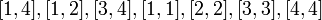$[1,4], [1,2], [3,4], [1,1], [2,2], [3,3], [4,4]$, exactly as for a one-dimensional segment tree. The node for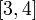$[3,4]$, for example, would itself be a tree on the boxes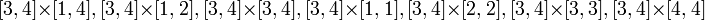$[3,4]\times[1,4], [3,4]\times[1,2], [3,4]\times[3,4], [3,4]\times[1,1], [3,4]\times[2,2], [3,4]\times[3,3], [3,4]\times[4,4]$.

The application of higher-dimensional segment trees to geometrical problems is more complex and is discussed in a separate article.

## Lazy propagation

For certain types of segment trees, range updates are possible. Consider, for example, a variation in the range minimum query problem in which we can not only update individual elements but also request that every element in a given range be set to the same specified value. This would be known as a range update. Lazy propagation is a technique that allows range updates to be carried out with the same asymptotic time complexity,$\mathcal{O}(\lg N)$, as individual updates.

The technique works as follows: each node contains an additional lazy field, which will be used for temporary storage. When this field is not being used, its value will be set to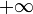$+\infty$. When updating a range, we select the same set of nodes that we would select if querying that range, and update their lazy fields to the desired value (that is, we set them to the new value if the new value is lower), except for leaf nodes, whose values are immediately set to the new value. When a query or update operation requires us to access a proper descendant of any node whose lazy field is set (i.e. not$+\infty$), we "push" the lazy field onto the two children: that is, we update the children's lazy fields according to the parent's and reset the parent's to$+\infty$. If, however, we access the node without accessing any of its proper descendants, and the lazy field is set, we simply use the value of the lazy field as the minimum for that node's associated interval.